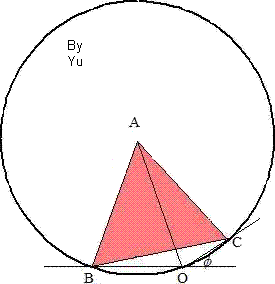## Monday, March 29, 2010

### Fox 266 - Solutions

Solutions of Fox 266 by Joe, Bleaug, Yu, and Giannno are similar. There is a different geometric solution by Binary Descartes, we should add it in the following days.

Bleaug:
No words needed. (Hey y not? say somethin' :)Yu:Given phi=30°, then m(BOC) = 150° which is 1/2 of reflex m(BAC).
.:B, O and C are on the same circle centred at A. Hence AB = AO.

Giannno:Extend CA such a way that AD=AC. Then m(DBC)=90° (since BA=DC/2 =AD=AC) and m(D)=30° and since m(BOC)=150° we get DBOC cyclic quadrilateral while A is the center of the circle. Hence AB=AO=AC radii of the same circle.
Migue: